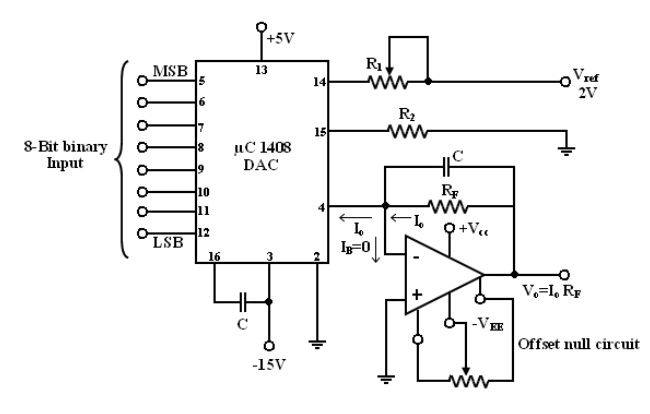Home | | Linear Integrated Circuits | Current to Voltage Converter (I-V)

# Current to Voltage Converter (I-V)

1. Sensitivity of the I – V converter 2. Applications of V-I converter with Floating Load 3. Applications of I – V Converter 4. DAC using I – V converter

Current to Voltage Converter (I –V):1.   Open – loop gain a of the op-amp is very large.  V1»V2    @@@@ `1a

2.   Input impedance of the op-amp is very high. (i.e) the currents entering into the 2 input terminals is very small. IB=IB2

1. Sensitivity of the I – V converter:

1.        The output voltage V0  = -RFIin.

2.        Hence the gain of this converter is equal to -RF. The magnitude of the gain (i.e) is also called as sensitivity of I to V converter.

3.        The amount of change in output volt ∆V0 for a given change in the input current ∆Iin is decide by the sensitivity of I-V converter.

4.        By keeping RF   variable, it is possible to vary the sensitivity as per the requirements.

2. Applications of V-I converter with Floating Load:

1. Diode Match finder:In some applications, it is necessary to have matched diodes with equal voltage drops at a particular value of diode current. The circuit can be used in finding matched diodes and is obtained from fig (V-I converter with floating load) by replacing RL with a diode. When the switch is in position 1: (Diode Match Finder) Rectifierr diode (IN 4001) is placed in the f/b loop, the current through this loop is set by input voltage Vin and Resistor R1 . For Vin = 1V and R1 = 100Ω, the current through this

I0 = Vin/R1 = 1/100 = 10mA.

As long as V0 and R1 constant, I0 will be constant. The Voltage drop across the diode can be found either by measuring the volt across it or o/p voltage. The output voltage is equal to (Vin + VD ) V0 = Vin + VD . To avoid an error in output voltage the op-amp should be initially nulled. Thus the matched diodes can be found by connecting diodes one after another in the feedback path and measuring voltage across them.

2. Zener diode Tester:

(When the switch position 2)

when the switch is in position 2, the circuit becomes a zener diode tester. The circuit can be used to find the breakdown voltage of zener diodes. The zener current is set at a constant value by Vin and R1. If this current is larger than the knee current (IZK ) of the zener, the zenerr blocks (Vz ) volts.

For Ex:

IZK = 1mA , VZ = 6.2V, Vin = 1v , R1 = 100Ω Since the current through the zener is , I0 = Vin/R1 = 1/100 =10mA > IZK the voltage across the zener will be approximately equal to 6.2V.

3. When the switch is in position 3: (LED)

The circuit becomes a LED when the switch is in position 3. LED current is set at a constant value by Vin and R1. LEDs can be tested for brightness one after another at this current. Matched LEDs with equal brightness at a specific value of current are useful as indicates and display devices in digital applications.

3. Applications of I – V Converter:One of the most common use of the current to voltage converter is

1.        Digital to analog Converter (DAC)

2.        Sensing current through Photodetector. Such as photocell, photodiodes and photovoltaic cells.

Photoconductive devices produce a current that is proportional to an incident energy or light (i.e) It can be used to detect the light.

4. DAC using I – V converter:

It shows a combination of a DAC and current to voltage converter. The 8 digit binary signal is the input to the DAC and V0 is the corresponding analog output of the current to voltage converter. The outputu of the DAC is current I0, the value of which depends on the logic state (0 or 1), of the binary inputs as indicated by the following eqn.

This means I0 is zero when all inputs are logic 0.

I0 is max when all inputs are logic 1.

The variations in I0 can be converted into a desired o/p voltage range by selecting a proper value for RF. since, V0 = I0 RF

Where I0 is given by eqn (1). It is common to parallel RF with capacitance C to minimize the overshoot. In the fig the o/p voltage of the current to voltage converter is positive because the direction of input current I0 is opposite to that in the basic I – V Converter.

Detecting current through photosensitive devices:Photocells, photodiodes, photovoltaic cells give an output curren that depends on the intensity of light and independent of the load. The current through this devices can be converted to voltage by I – V converter and it can be used as a measure of the amount of light. In this fig photocell is connected to the I – V Converter. Photocell is a passive transducer, it requires an external dc voltage(Vdc). The dc voltage can be eliminated if a photovoltaic cell is used instead of a photocell. The Photovoltaic Cell is a semiconductor device that converts the radiant energy to electrical power. It is a self generating circuit because it doesnot require dc voltage externally. Ex of Photovoltaic Cell : used in space applications and watches.

Study Material, Lecturing Notes, Assignment, Reference, Wiki description explanation, brief detail
Linear Integrated Ciruits : Application of Op-Amp : Current to Voltage Converter (I-V) |